# Wave Behavior Lab Rotation: Day 2

47 teachers like this lesson
Print Lesson

## Objective

Students will be able to create simple mathematical representations of waves and identify characteristic properties of waves.

#### Big Idea

Students go through a series of hands-on lab stations that engage students in not only identifying the properties of waves, but in creating mathematical representations as well!

## Introduction and Connections to the NGSS and Common Core

This is the second day of a lesson designed to provide students the opportunity to travel through a series of lab stations that connect to the following NGSS and Common Core Standards:

MS-PS4-1  Use mathematical representation to describe a simple model for waves that includes how the amplitude of a wave is related to the energy in a wave.

MS-PS-4-2  Develop and use a model to describe that waves are reflected, absorbed, or transmitted through various materials.

CCSS.ELA Reading: Science & Technical Subjects Integrate quantitative or technical information expressed in words in a text with a version of that information expressed visually (e.g., in a flowchart, diagram, model, graph, or table).

CCSS.7.Ratios and Proportional Relationships 2 Recognize and represent proportional relationships between quantities.

CCSS.Mathematical Practice 2 Reason abstractly and quantitatively.

CCSS.ELA-LITERACY.WHST.6-8.2.A  Introduce a topic clearly, previewing what is to follow; organize ideas, concepts, and information into broader categories as appropriate to achieving purpose; include formatting (e.g., headings), graphics (e.g., charts, tables), and multimedia when useful to aiding comprehension.

At this point, students have had multiple experiences engaging with each of these standards in previous lessons.  They have created models representing energy and amplitude and have explored the relationship between frequency, wavelength, and pitch using slinkies.  Moreover, they have created models of transmission, absorption, and reflection.  This series of lab stations is therefore an extremely independent activity for students providing them with more interactions with the standards.  Therefore, it is a great lesson to focus on students that have not been demonstrating mastery on specific topics and help them make connections that they have not yet previously made.

In this lab rotation, students continue to create models that explain phenomena (SP2).  In addition, students utilize the Crosscutting Concept of Patterns by using graphs to identify patterns and relationships between variables such as frequency and wavelength.  However, one new aspect that we begin to discuss is the mathematical representation of what a wave looks like and how proportions and scale can effect the graph of a wave.  Thus, this lesson also addresses the Crosscutting Concept of Scale, Proportion, and Quantity.  This explains that "in considering phenomena, it is critical to recognize how changes in scale, proportion, or quantity affect a system’s structure or performance."

On Day 1 of the lesson, students are introduced to the idea of how a wave can be graphed in a coordinate plane on a Displacement vs. Time/Distance plot.  In this lab, students have to create graphs that demonstrate general relationships of both transverse and longitudinal waves in order to develop their mathematical and conceptual thinking (SP5).  On this second day of instruction, students are introduced to graphing a wave based on specific numbers instead of general relationships and begin manipulating the graphs of waves to demonstrate a proportional understanding of wave relationships.

## Connecting to the EQ: What are you going to be learning today? - Day 2

10 minutes

Begin the class by saying, "What are you going to be learning today?"  Students should respond by referring to the NGSS Essential Question which I have posted on the board and on their Unit Plan, "What are the characteristic properties of waves and how can they be used?".  Ask the students to refer to the Light Bulb sheet that they received yesterday and to take 5 minutes to add any information they connected to in the labs the previous day to either of the light bulb pages.   Then, discuss as a class and have the students add information to their light bulb that they did not previously have.

## Mini Lesson - Day 2

25 minutes

Below is one way the mini lesson could unfold.  Keep in mind that students had exposure and experience with the definitions and relationships between wave properties.  Also, in the previous lesson they were introduced to the idea of the variables that might be labeled on the x and y axis in the graph of a wave.

Teacher:  Yesterday, you began talking about how scientists use math to show relationships and that you introduced ways that waves can be graphed.  We discussed general ideas about how waves can be graphed.  Today, we are going to graph waves based on given numbers.

In my classroom, I happen to have a "drop down" graph attached to my white board.  If you do not have one, but have a document camera or computer/projector, I would highly recommend using some form of media that can show graph paper so that you can write on it as you teach.

Teacher:  I am trying to remember from yesterday what labels we mentioned could be used on a graph of a wave.  What were those again?

Student Volunteer: Displacement should be placed on the y axis and that either time or distance should be placed on the x axis.

Write "Displacement" on the y axis.

Teacher:  How would I know whether I would use 'Time' or 'Distance' on the x axis?

Student: If you are graphing wavelength, it would be labeled 'Distance" but if you are graphing frequency, it would be labeled 'Time'.

Teacher:  What if I wanted to graph a wave that had an amplitude of 4 and a wavelength of 6?  What should I label the x axis and why?

Student:  Distance because wavelength is the distance from crest to crest.

Label the x axis with the word "Distance".  Place this label well below the x axis as you will be drawing a wave that will go below the x axis.

Teacher:  What do you know about the wave I am graphing?  What does that wave look like?

Student:  It is 4 units tall because it has an amplitude of 4.

Teacher:  So, I should make the crest 2 units above the x axis and 2 units below the x axis?  (Show with your hands on the projection.)

Student:  No, amplitude is a measure of the wave from a line through the middle of the wave to a crest.

Teacher:  Oh!  So, each crest should be 4 above the x axis and each trough should be 4 below!  That makes sense!  What else do I need to know about what the wave looks like?

Student: It has a wavelength of 6.

Teacher:  What does that mean?

Student:  The distance from crest to crest or trough to trough is 6.

Teacher:  Now, I know that the graphs of waves look like transverse waves. So, I know that I am going to be drawing a line that goes up and down.  I want to make sure I have some reference points so I can draw that line effectively.  What was the amplitude again?

Student:  4.

Teacher:  Okay, I am going to put a reference mark at 4.  It is not an exact point, just a reference mark to help me draw a better curved line.  This reference point is at the top of the wave, so it must be the crest.  How do I know where the next crest will be?

Student:  6 away.

Teacher:  How do you know?

Student:  The wavelength is from one crest to the next crest.  The wavelength here is 6.

Teacher:  Great!  I am going to put another reference point 6 units to the right of the first reference point.  Then, I am going to add one more crest 6 units away from there.  I know a wave has crest and troughs.  Where should I put the troughs?

Student:  Half way between the crests.

Teacher:  Oh...the distance between the crests is 6.  Halfway in between would be 3.  How far down should I go?

Student:  4!  The amplitude is 4.

Count over 3 spaces from each crest reference point and place a dot 4 spaced below the x axis.Teacher:  Now that I have some reference points, I can draw my wave.

Starting at the first crest, draw a transverse wave that connects the reference points.Teacher:  Awesome!  I see that this wave has an amplitude of 4 (it rises above the x axis 4) and a wavelength of 6 (the distance from crest to crest).  Now, what if I said, "Draw another graph of a wave on this same graph that had 1/2 the amplitude but 2x the wavelength."  How would you begin graphing this?

Student:  I would divide the amplitude by 2.

Teacher:  Oh, I see. Because it is 1/2 the amplitude we can divide 4 by 2.  What would the new amplitude be?

Student:  2.

Teacher:  Great!  What about the wavelength?  What would we need to figure out next?

Student:  Multiple the wavelength of 6 by 2.  So the wavelength would be 12.

Teacher:  Excellent!  If I am looking for something twice as big, I would multiply by 2.

Graph the wave by placing a reference point 2 up on the y axis and then counting over 12 spaces and putting another reference point.

Teacher:  Now I have my crests.  Where should I put my troughs?

Student:  They are halfway in between, so 12/2 = 6 units.  And they should go 2 units below the x axis.

Draw the trough reference points.  And draw the new wave.  Ask students to notice how the new wave is half the amplitude but the wavelength is twice as long.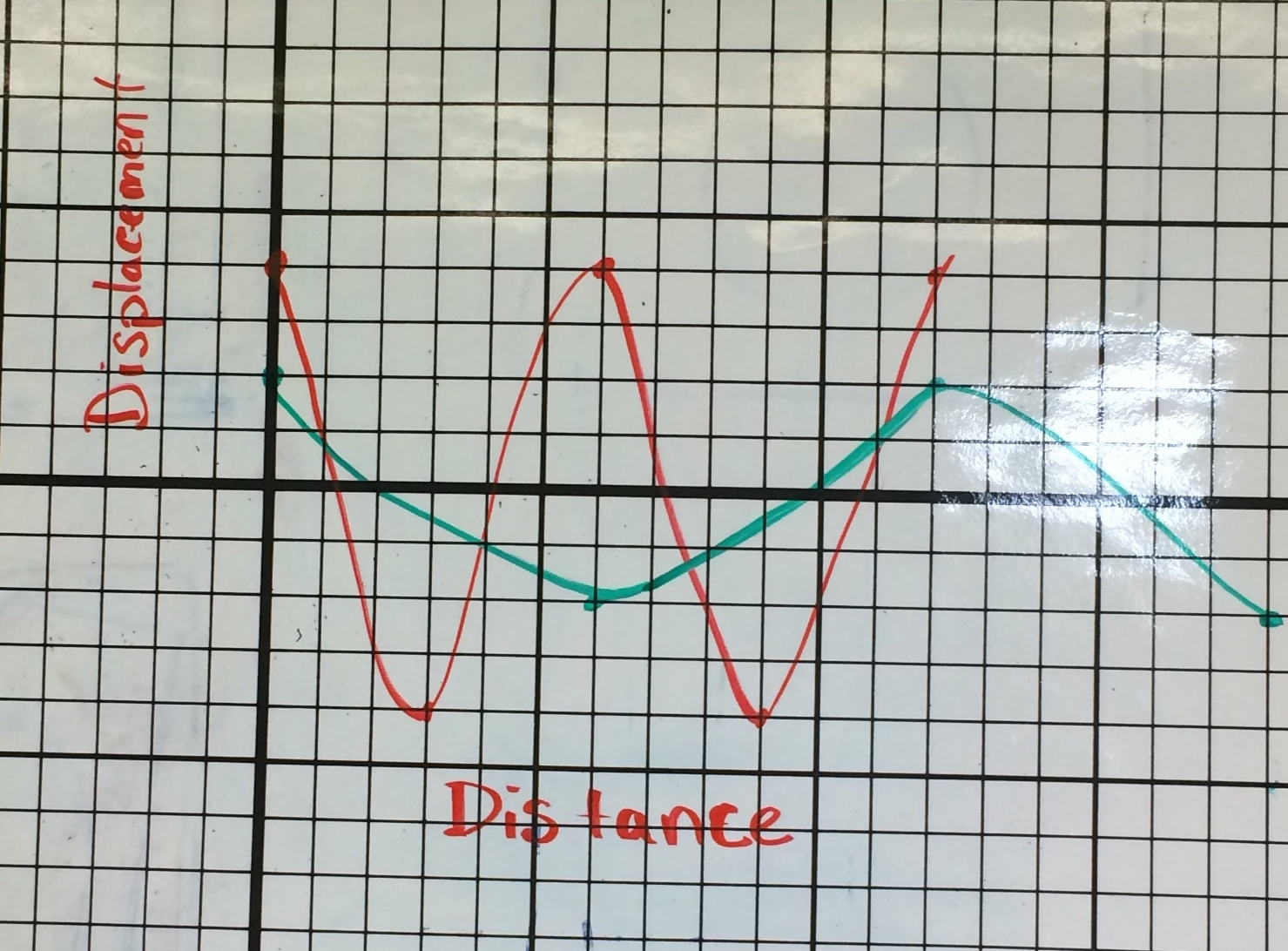Next, erase the new wave line that you just drew, but leave the original (amplitude of 4, wavelength of 6) line.  Erase the x axis label, "Distance" as well.

Teacher:  How could I add a line that is twice the amplitude and twice the frequency of this line?

Student:  You could multiply the amplitude by 2.  4 x 2 - 8.  The new amplitude would be 8.

Teacher:  Ahh.  That make sense.  Did anyone notice that I erased the x axis label, "Distance".  Why did I do that?

Student:  Now we are representing frequency which we should label with time.

Add Time as the label to the x axis.

Teacher:  As I am looking at this graph, I am kind of stuck on how to graph frequency.  The thing I feel most comfortable measuring is wavelength.  Is there any relationship between frequency and wavelength that could help me decide how to create this graph?

Student:  As frequency increases, wavelength decreases.

Teacher:  Oh yeah, frequency and wavelength are inversely proportional.  As frequency increases (I put one thumb up in the air), wavelength decreases (I leave the thumb up and place the other thumb down.)  So, if I need to make a wave that has a frequency that is double this original wave, what should I do?

Student:  Divide the wavelength by 2.  The wavelength right now is 6, 1/2 the wavelength would be 3.

Teacher:  I am trying to figure out why you wanted to divide the wavelength when we need to multiply the frequency by 2.  Could everyone picture making a transverse slinky wave? (Wait time.)  Now, picture a slinky wave that is twice the frequency.  What is the wavelength like?

Student:  It's shorter!

Teacher:  How much shorter?

Student:  Half!

Teacher:  So that means we need to divide the wavelength by 2!  The wavelength will be 3.

Draw reference points on the graph.  Crest reference points should all be 2 up and 3 units apart.  Connect the reference points so the students can see the end product.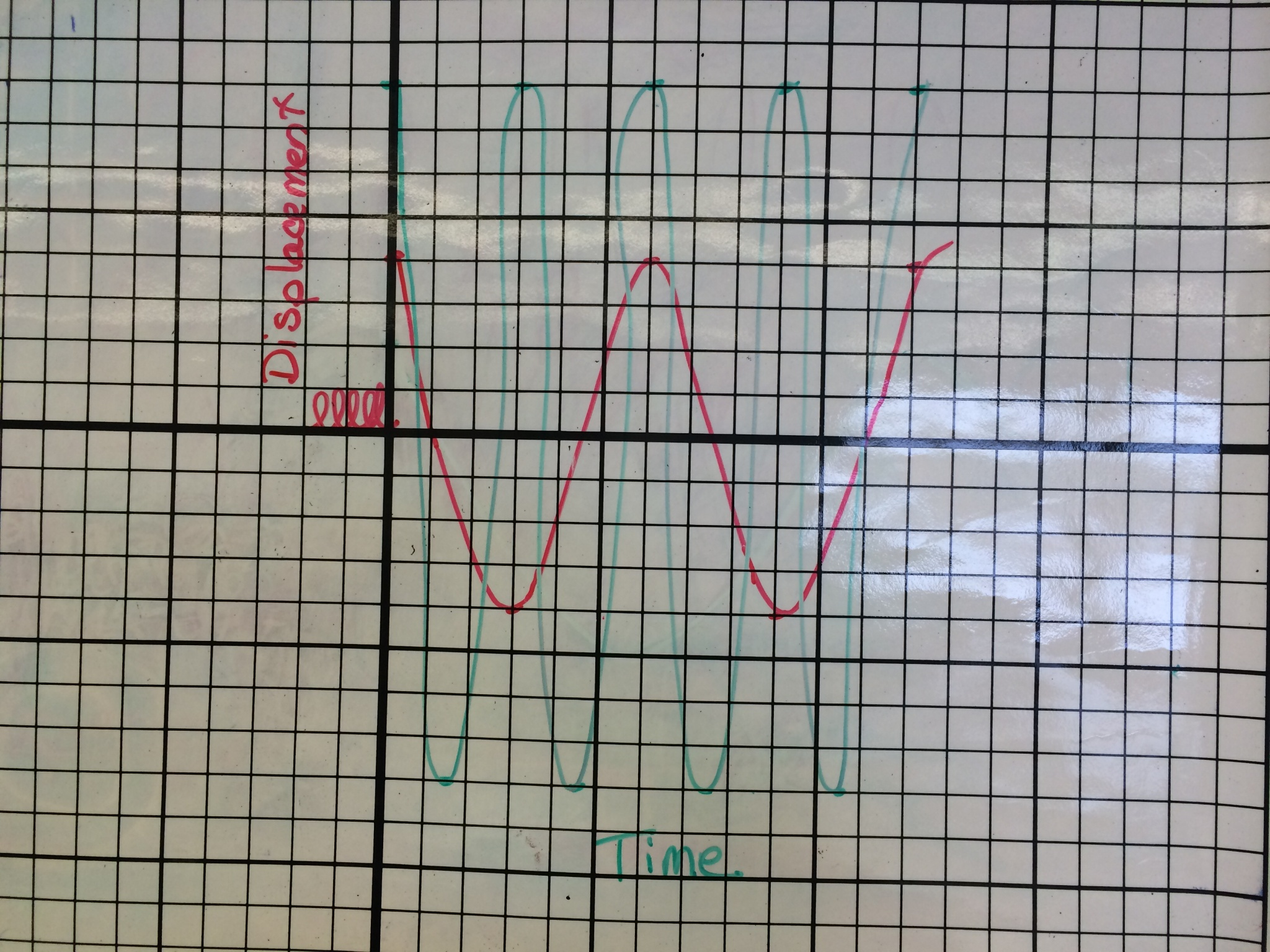Have the students work on and complete the Graphing of Wave worksheet.  Let students know that this is their first attempt at graphing these and that you are open for questions and would love to help.  Emphasize that this is their first practice and will not count "against" their grade. Ease students fears by saying something like, "This is your first attempt in learning with graphing waves.  You may not have mastered them yet, celebrate your initial struggles by asking for help!"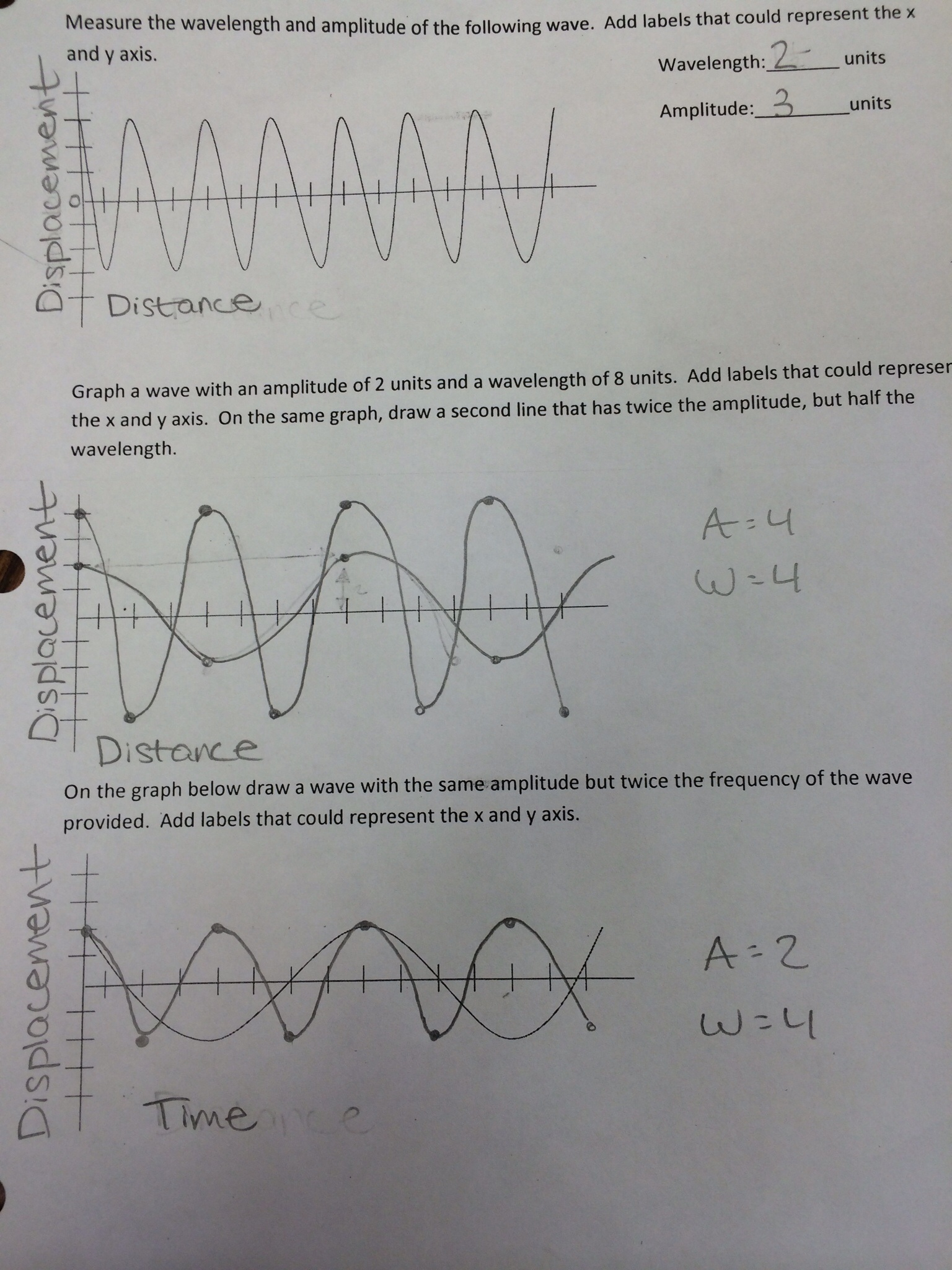There are a few important things to notice about the student work shown above.  In the first graph, notice that the student labeled both the x and y axes.  In addition, as the question asks to measure wavelength, the student labeled the x axis "Distance".  In the second graph, the student again labels the axes appropriately.  Moreover, with the writing on the side, it is clear that this student manipulated the amplitude and wavelength numbers by using proportions rather than trying to "eyeball it".  I have some students that want to start drawing the second line without calculating the new amplitude and wavelength numbers by multiplying and dividing.  Writing the new measurements down can provide the student with a clear path and direction for drawing their wave.  For the third graph, notice that the student labeled the x axis "Time" instead of "Distance" as frequency must have a time reference.  (I also love that the student caught themselves and erased "Distance" and replaced it with "Time"!)  Again in the third graph, the student made a clear plan manipulating the amplitude and wavelength numbers proportionally.

## Wave Behavior Lab Rotation - Day 2

35 minutes

Students should continue with the lab stations.  A couple of things to remind the students about their Behavior of Waves Lab Document:  (For more information about materials and set up of each lab station, see the Wave Behavior Lab Rotation Day 1 lesson plan.)

1.  Evidence is key!  Be sure to provide evidence that you observe in the conclusions you make, answers you write, and diagrams you draw.

2.  At the "Traveling Sound" station, the purpose* of the diagrams are different.  Take time to analyze the purpose of each diagram and tell students to ask for clarification if they have questions.

*The first diagram on the "Traveling Sound" station asks students to show how the different types of strings conducted the sound differently.  The second diagram explains how sound moves from the hanger through the string.

Evaluating Student Work While They Work

Below I have included some examples of student work from each station with critical information on what to look for, and what students often give us.  As students work their way around the stations, look for these things as talking points or teaching moments with students.  Don't wait to provide feedback until they turn it in.  Engage with them about their responses as they rotate!

## Student Work and Discussion Points: Stations 1 and 2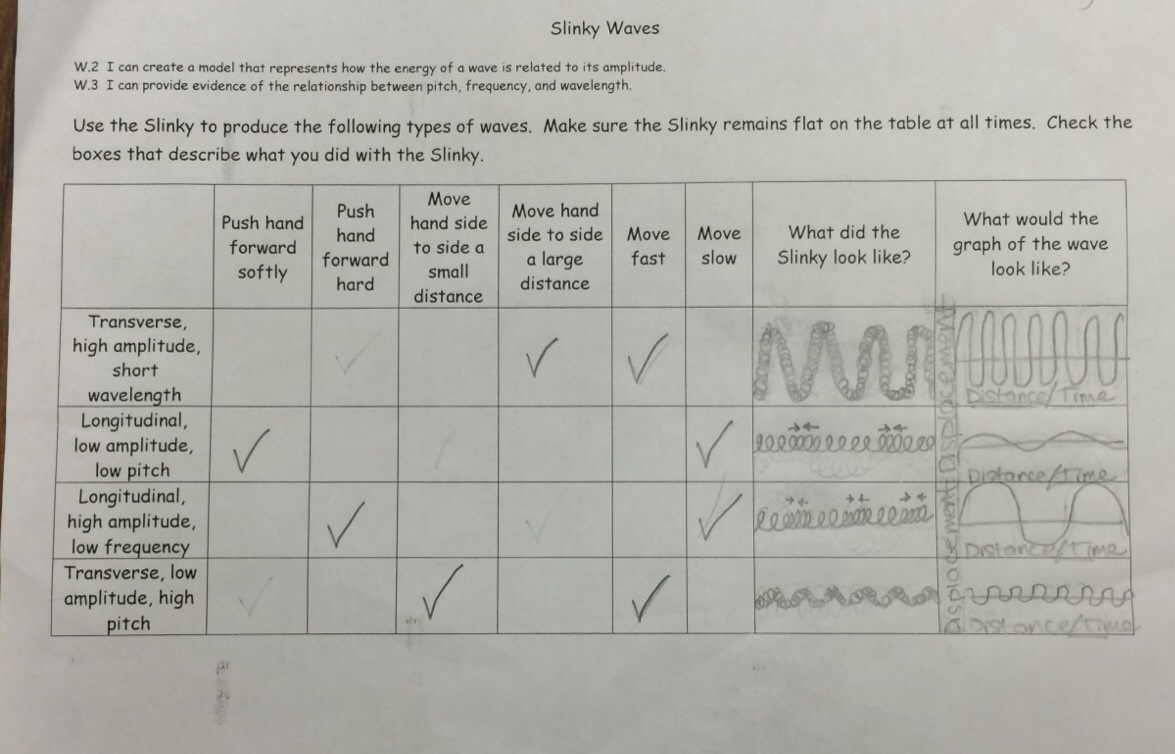Here the student has identified correctly how to create each wave type with a slinky. In addition, they have drawn what the slinky looks like so that the properties of the waves are clear.  For example, the high amplitude transverse wave in the first row is taller than the low amplitude transverse wave in the bottom row.  In addition, the student has included the x and y axis on her graphs and has included labels.  As you walk around and check in with students, a really important connection to ask students about is what they notice about how the slinky looks compared to the graph.  Recognition that transverse waves look similar to their graphs but longitudinal waves look different is a common challenge for middle school students.One check for understanding is if students are recognizing that the rows that mention "frequency" in the wave description have "Time" on the x axis, while those referencing wavelength have "Distance" on the x axis.  The student above shows this understanding.

Traveling Sound

Diagram #1, Student #1:Diagram #1, Student #2: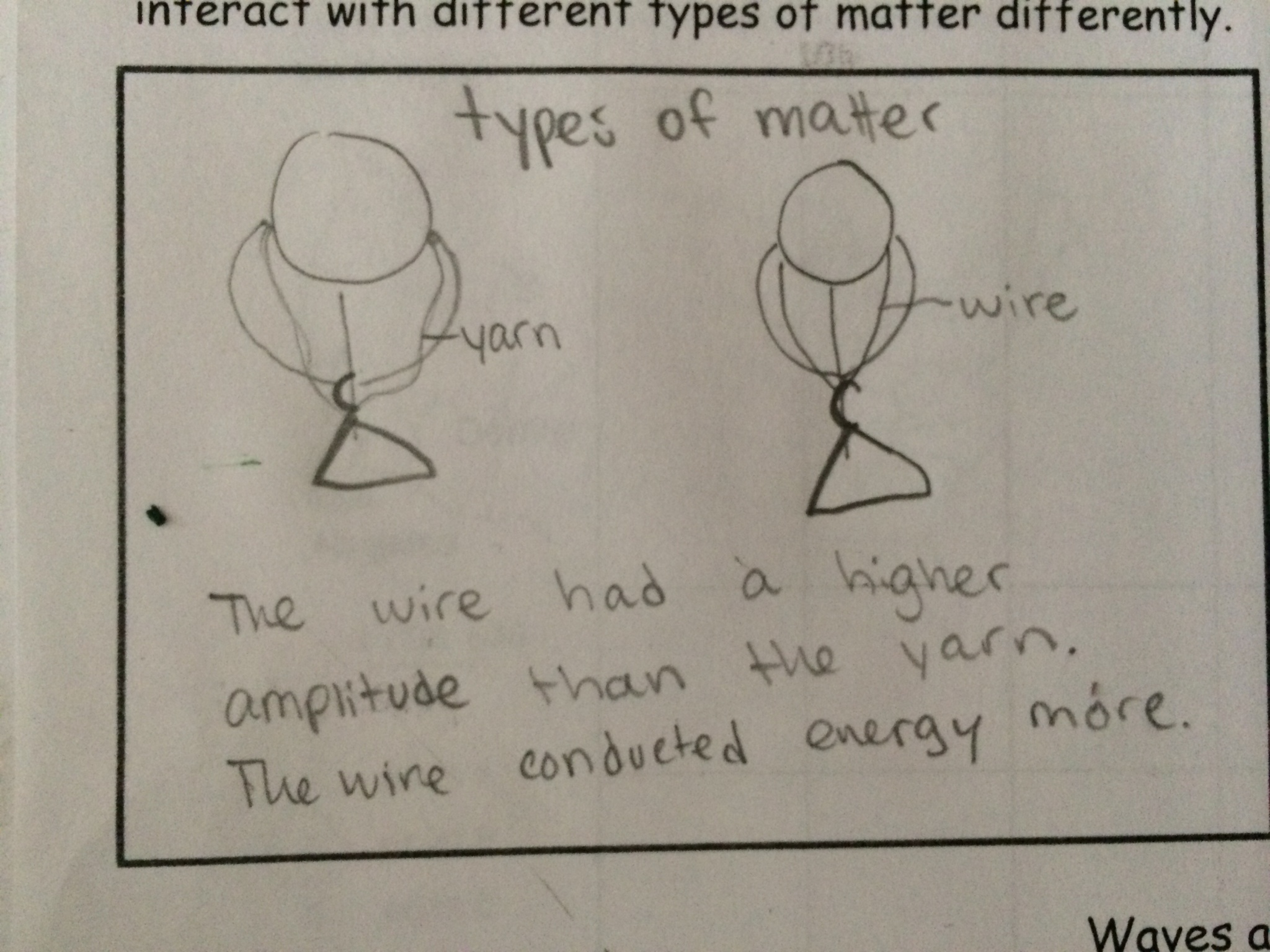Students may key in on different vocabulary to explain phenomena.  It is one thing I enjoy about creating diagrams and models.  Students may show understanding in different ways.  Student #1 above explained that the yarn "absorbed" more of the waves, while the Student #2 above explained that the wire "conducted the energy more".No matter how many times and different ways you may teach that waves do not transmit or transfer matter, students have a misconception that it does.  If I asked this student specifically if waves "transmit matter", he would say, "No, only energy."  However, when put in situations to write about waves, it is common for students to say that it does.  The student above says that the sound, "conducted matter."  Watch out for misconceptions! They are great teaching moments!

Diagram #2. Student #1:Diagram #2, Student #2: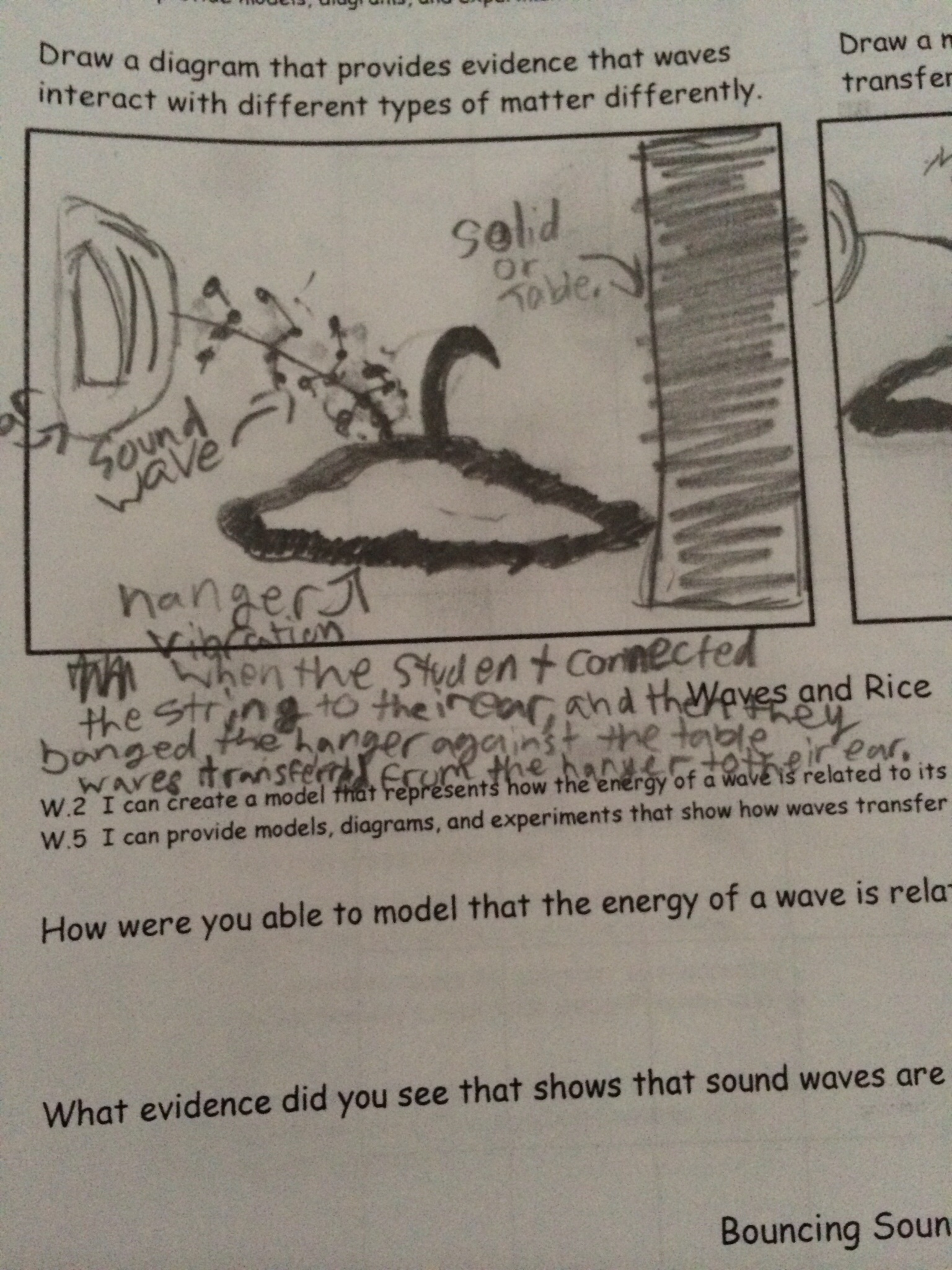While both of these students showed how sound moved in different ways, both students mentioned vibrations as the source of the sound and mentioned compressions in the way that the sound traveled.

## Student Work and Discussion Points: Stations 3, 4, and 5

Waves and RiceIn the first question at the "Waves and Rice" station, many students forget to use key vocabulary and connect to the purpose of the question.  Often times, they verbalize it well, but do not communicate it clearly when writing.  For example, the student above references amplitude and the idea that the rice jumped as evidence of that.  However, they did not mention energy at all.  When demonstrating a relationship, it is important to refer to both variables.

Bouncing Sound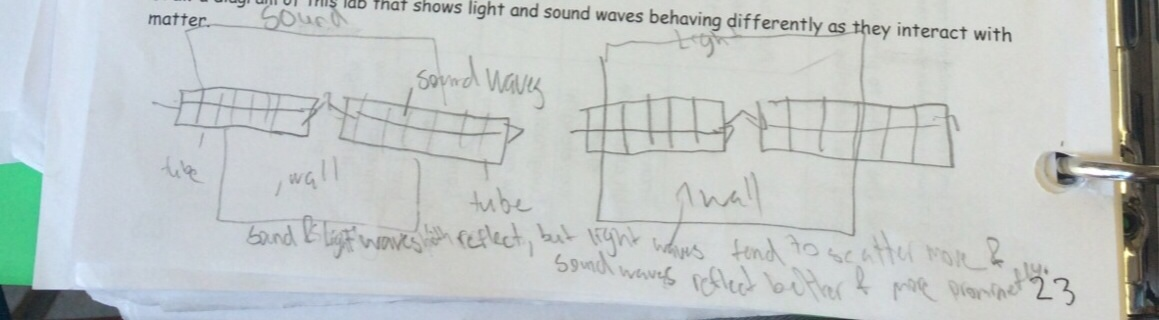The idea behind this station is that light and sound waves interact similarly and differently with matter.  This student explains that both light and sound reflect of the wall, but that more of the light is absorbed/scattered/etc. than the sound.  One misconception here is that students think the light doesn't reflect at all.  It is important that they realize that if they can see the light on the wall, some of the light is reflecting back to their eyes.  I do follow up by saying, "I agree with what you are leading to.  If I was to perform this same activity against a mirror instead of a wall, there would be a difference in the reflection of the light."

Refraction Cells and PrismsOften students can "forget" to add evidence here.  They instead may write, "color" or "prism".  It is key that they identify evidence.  I always direct them to what they are supposed to be learning, or the skill, listed under the title of the lab.  I ask them to review what the "key verbs" are in the skill that tells them what they have to be able to do with the vocabulary in the skill.  This skill says, "provide evidence."  The student above provides evidence in each case.  On a side note, one common piece of evidence that students use for absorption that is different than what this student wrote is that when the laser pointer was shined on the prism or cell, there was "less" light showing on the opposite side.

## Closure and Student Work

15 minutes

To close the lesson, ask the students to have their labs in front of them, turn to a partner, and answer the following:

1.  What evidence can you cite that different types of waves interact with matter in different ways?
2. How can you create a mathematical representation of wave properties?

Remind students that as scientists we cite our data and text.  Thus, as students share their examples, they should turn to the lab document and actually point out and reference information they have written in their labs.  Just as they cited the paragraph and line of the text in the "How do satellites work?" article during Day 1 of this lesson, they should indicate their evidence and documentation in their lab documents as they share.  In addition, encourage students to add to their own writing, diagrams and evidence if they learn anything new from their peers.